## Example Questions

### Example Question #21 : Decimals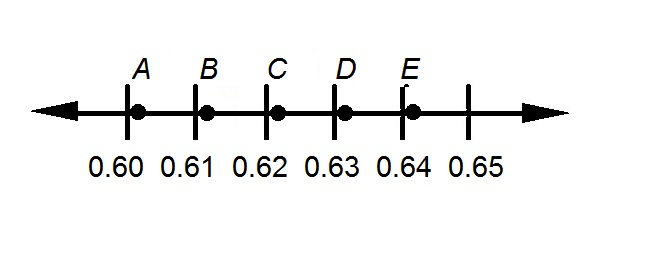In the above number line, which point comes closest to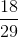?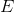Explanation:

Divide numerator 18 by denominator 29 to find the decimal equivalent of the fraction: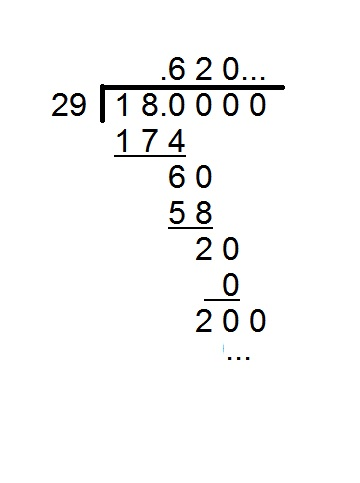The result is slightly higher than 0.62 - the pointon the number line.

### Example Question #1 : Decimals With Fractions

Write 0.45 as a fraction.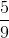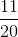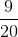Explanation:

.45 is equivalent to 45 out of 100, or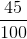.

Divide both the numerator and denominator by 5 to simplify the fraction:### Example Question #1 : Decimals With Fractions

A tub of food containspounds of vegetables,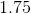pounds of lard, andpounds of sausage.  What is its total weight as an improper fraction?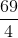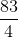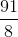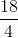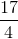Explanation:

To begin with, it is easiest just to add these decimals together using your calculator: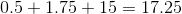Now, this is the same thing as: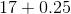We can rewrite this: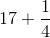To find this, you need to give the two numbers a common denominator: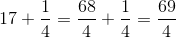### Example Question #1 : Decimals

What is the fractional equivalent of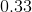?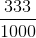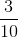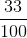Explanation:

In decimal formis said 33 hundredths.

This is equal to.

This fraction cannot be reduced any further therefore it is in its final answer form.

### Example Question #1251 : Sat Mathematics

Convert the decimal to fraction form and reduce it to its simplest form.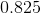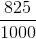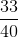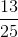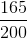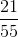Explanation:

In order to convertto a fraction, you would first begin with, because the decimal literally reads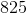thousandths. You can reduce bya few times or just begin by dividing both numbers byto get.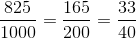### Example Question #1 : How To Square A Decimal

If all real values oflie between 0 and 1, which of the following is always greater than 1?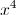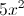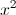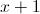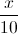Explanation:

Ifis greater than 0, then adding 1 towill make it greater than 1. Taking a number between 0 and 1 to a power results in a smaller number.

### Example Question #1 : How To Square A Decimal

Evaluate:

0.082

0.064

0.00064

0.64

0.0064

0.00064

0.0064

Explanation:

0.08 * 0.08

First square 8:

8 * 8 = 64

Then move the decimal four places to the left:

0.0064

### Example Question #1 : Basic Squaring / Square Roots

Find the square root of the following decimal: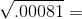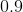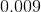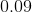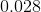Explanation:

The easiest way to find the square root of a fraction is to convert it into scientific notation.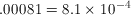The key is that the exponent in scientific notation has to be even for a square root because the square root of an exponent is diving it by two. The square root of 9 is 3, so the square root of 8.1 is a little bit less than 3, around 2.8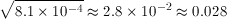### Example Question #1 : How To Find The Square Root Of A Decimal

Find the square root of the following decimal: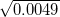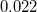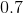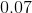Explanation:

To find the square root of this decimal we convert it into scientific notation.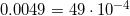Because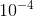has an even exponent, we can divide the exponenet by 2 to get its square root.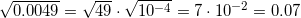### Example Question #1 : How To Find The Square Root Of A Decimal

Find the square root of the following decimal: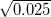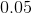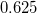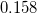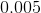Explanation:

This problem can be solve more easily by rewriting the decimal into scientific notation.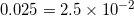Because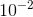has an even exponent, we can take the square root of it by dividing it by 2. The square root of 4 is 2, and the square root of 1 is 1, so the square root of 2.5 is less than 2 and greater than 1.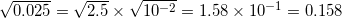### All SAT Math Resources